# User:Tohline/H Book

(diff) ← Older revision | Current revision (diff) | Newer revision → (diff)

Preface from the original version of this HyperText Book (H_Book):

November 18, 1994

Much of our present, basic understanding of the structure, stability, and dynamical evolution of individual stars, short-period binary star systems, and the gaseous disks that are associated with numerous types of stellar systems (including galaxies) is derived from an examination of the behavior of a specific set of coupled, partial differential equations. These equations — most of which also are heavily utilized in studies of continuum flows in terrestrial environments — are thought to govern the underlying physics of all macroscopic "fluid" systems in astronomy. Although relatively simple in form, they prove to be very rich in nature... <more>

# Context

• Global Energy Considerations

# Applications

## Spherically Symmetric Configurations

### Structure:

🎦 ⚜

Here we show how the set of principal governing equations (PGEs) can be solved to determine the equilibrium structure of spherically symmetric fluid configurations — such as individual, nonrotating stars or protostellar gas clouds. After supplementing the PGEs by specifying an equation of state of the fluid, the system of equations is usually solved by employing one of three techniques to obtain a "detailed force-balanced" model that provides the radius,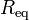$~R_\mathrm{eq}$, of the equilibrium configuration — given its mass,$~M$, and central pressure,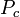$~P_c$, for example — as well as details regarding the internal radial profiles of the fluid density and fluid pressure. As our various discussions illustrate (see the table of contents, below), simply varying the power-law index in a polytropic equation of state gives rise to equilibrium configurations that have a wide variety of internal structural profiles.

If one is not particularly concerned about details regarding the distribution of matter within the equilibrium configuration, a good estimate of the size of the equilibrium system can be determined by assuming a uniform-density structure then identifying local extrema in the system's global free energy. In the astrophysics community, the mathematical relation that serves to define the properties of configurations that are associated with such free-energy extrema is often referred to as the scalar virial theorem<more>

In the following table, each green check mark identifies and provides a link to an H_Book chapter that presents a detailed discussion of the topic that is identified on the left — for example, the equilibirum structure of "isolated polytropes" or an "isothermal sphere embedded in an external medium." Mathematical models that provide full solutions to the PGEs, including details regarding the internal structural profiles of equilibrium configurations, are derived in chapters whose check marks fall under the column labeled "Detailed Force-Balance." Insight into the properties of equilibrium systems that is revealed via an analysis of a system's free-energy and the corresponding scalar virial theorem is presented in chapters whose check marks fall under the column labeled "Virial Equilibrium."

Solution Strategies:

Detailed Force-Balance
(Introduction)

Virial Equilibrium
(Introduction)

 Example Solutions: Uniform-density sphere Isolated … ✓ ✓ Embedded in an External Medium … ✓ ✓ Polytropes Isolated … ✓ ✓ Embedded in an External Medium … ✓ ✓   🎦 Isothermal sphere Isolated … ✓ ✓ Embedded in an External Medium (Bonnor-Ebert Sphere) … ✓ ✓ Zero-temperature White Dwarf — Overview Power-law density distribution — Overview Other Analytically Definable Models ✓ … BiPolytropes (also referred to as Composite Polytropes) Overview Overview Core-Envelope Structure with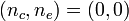$~(n_c,n_e) = (0,0)$ … ✓ ✓ Core-Envelope Structure with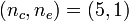$~(n_c,n_e) = (5,1)$ … ✓ ✓ Core-Envelope Structure with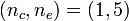$~(n_c,n_e) = (1,5)$ … ✓ … Core-Envelope Structure with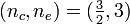$~(n_c,n_e) = (\tfrac{3}{2},3)$ … ✓ …

### Stability:

Suppose we want to study the stability of one of the spherically symmetric, equilibrium structures that has been described in the above "Structure" chapters. One approach is to solve an eigenvalue problem whose governing second-order ODE — for example, the Linear Adiabatic Wave Equation (LAWE) — comes directly from perturbing the equilibrium model and a linearization of the overarching set of principal governing equations. In the set of chapters that is identified in the upper-left quadrant of the following table of content, we explain how the relevant wave equation for self-gravitating systems is derived from either a Lagrangian perspective or an Eulerian perspective, and how it resembles the equation that describes the propagation of sound waves in terrestrial fluids. Another approach, which is described by the chapter(s) in the upper-right quadrant of the table, builds on a free-energy analysis of self-gravitating systems: If an extremum in the free energy is identified as a local energy minimum, then the identified equilibrium configuration is stable; but if the extremum is a local energy maximum, then the equilibrium configuration is unstable.

[This paragraph ultimately should be moved to a "more …" page] Text written in green has been taken directly from the introductory paragraphs of G. S. Bisnovatyi-Kogan & S. I. Blinnikov (1974). Three different approaches are used in the study of hydrodynamical stability of stars and other gravitating objects …   The first approach is based on the use of the equations of small oscillations. In that case the problem is reduced to a search for the solution of the boundary-value problem of the Stourme-Liuville type for the linearised system of equations of small oscillations. The solutions consist of a set of eigenfrequencies and eigenfunctions. This approach is subject to great numerical difficulties and has been carried through successfully only for the simplest case[s]. For spherically symmetric, Newtonian systems, see, for example, Schwarzschild (1941) or Ledoux & Walraven (1958). Second, one can derive a variational principle from the equations of small oscillations. This principle replaces the straightforward solution of these equations: In the context of rotating Newtonian systems, see, for example, Clement (1964), Chandrasekhar & Lebovitz (1968), Lynden-Bell and Ostriker (1967), or Schutz (1972). With the aid of the variational principle, the problem is reduced to the search of the best trial functions; this leads to approximate eigenvalues of oscillations. In spite of the simplifications introduced by the use of the variational principle and by not solving the equations of motion exactly, the problem still remains complicated … The third approach is what we have referred to as a free-energy analysis. When this method is used, it is not necessary to use the equations of small oscillations but, instead, the functional expression for the total energy of the momentarily stationary (but not necessarily in equilibrium) star is sufficient. The condition that the first variation of the energy vanishes, determines the state of equilibrium of the star and the positiveness of a second variation indicates stability. For non rotating systems having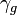$~\gamma_g$ near 4/3, see, for example, Fowler (1964) or Zeldovich & Novikov (Soviet journal, 1965).

If one wants to know from a stability analysis the answer to only one question — whether the model is stable or not — then the most straightforward procedure is to use the third, static method (Zeldovich 1963; Dmitrie & Kholin 1963). For the application of this method, one needs to construct only equilibrium, stationary models, with no further calculation. Generally the static analysis gives no information about the shape of the modes of oscillation, but, in the vicinity of critical points, where instability sets in, this method makes it possible to find the eigenfunction of the mode which becomes unstable at the critical point.

In the lower portion of the following table of content, links are provided to chapters in which one of these primary solution strategies is employed to analyze the stability of specific equilibrium models.

 Solution Strategy Assuming Spherical Symmetry: Ledoux's Variational Principle Early Chatting with Kundan Kadam Example Solutions: Uniform-density sphere Isolated Polytropes Setup n = 1:  Attempt at Formulating an Analytic Solution n = 3:  Numerical Solution to compare with M. Schwarzschild (1941) n = 5:  Attempt at Formulating an Analytic Solution Radial Oscillations in, and Stability of, Pressure-Truncated Configurations (Overview) Pressure-Truncated Isothermal Spheres Bounded and Composite Polytropes Bipolytropes having$~(n_c, n_e) = (0, 0)$ Overview Initial set of chapters that used incorrect interface matching condition One Analytically Specified Eigenvector for a Bipolytrope having$~(n_c, n_e) = (0, 0)$ Initial Detailed Derivation Additional Analytically Specified Eigenvectors Numerically Determined Eigenvectors Free-Energy Stability Analysis Compare Eigenvector and Free-Energy Analyses Also to be studied: Kimura (1981, PASJapan, 33, 299)

### Dynamics:

 Spherical Collapse: Free Fall Homologous Collapse Collapse of Stellar Cores with n = 3 — investigation by Goldreich & Weber (1980, ApJ, 238, 991) Generalization to Arbitrary Polytropic Index

## Two-Dimensional Configurations

### Structure:

 Solution Strategies Preference for Cylindrical Coordinates Axisymmetric Configurations (Part I) Axisymmetric Configurations (Part II) — including, Simple Rotation Profiles Infinitesimally Thin, Nonaxisymmetric Configurations Self-Consistent Field Technique Uniform-Density & Centrally Condensed Structures: Toroidal Structures: Papaloizou-Pringle (massless) Tori   🎦 Self-gravitating, Isothermal Rings Self-gravitating, Polytropic Tori Using Toroidal Coordinates to Determine the Gravitational Potential   🎦 Nonaxisymmetric Disks & Annuli: Infinitesimally Thin, Nonaxisymmetric Disk

### Stability:

 Solution Strategy Preference for Cylindrical Coordinates Centrally-condensed Structures: Rotating, Supermassive Stars Toroidal Structures: Papaloizou-Pringle (Massless) Tori Self-gravitating, Polytropic Tori

## Three-Dimensional Configurations

### Structure:

 Solution Strategies Example Solutions: Ellipsoidal Figures of Equilibrium Compressible Analogs of Riemann Ellipsoids